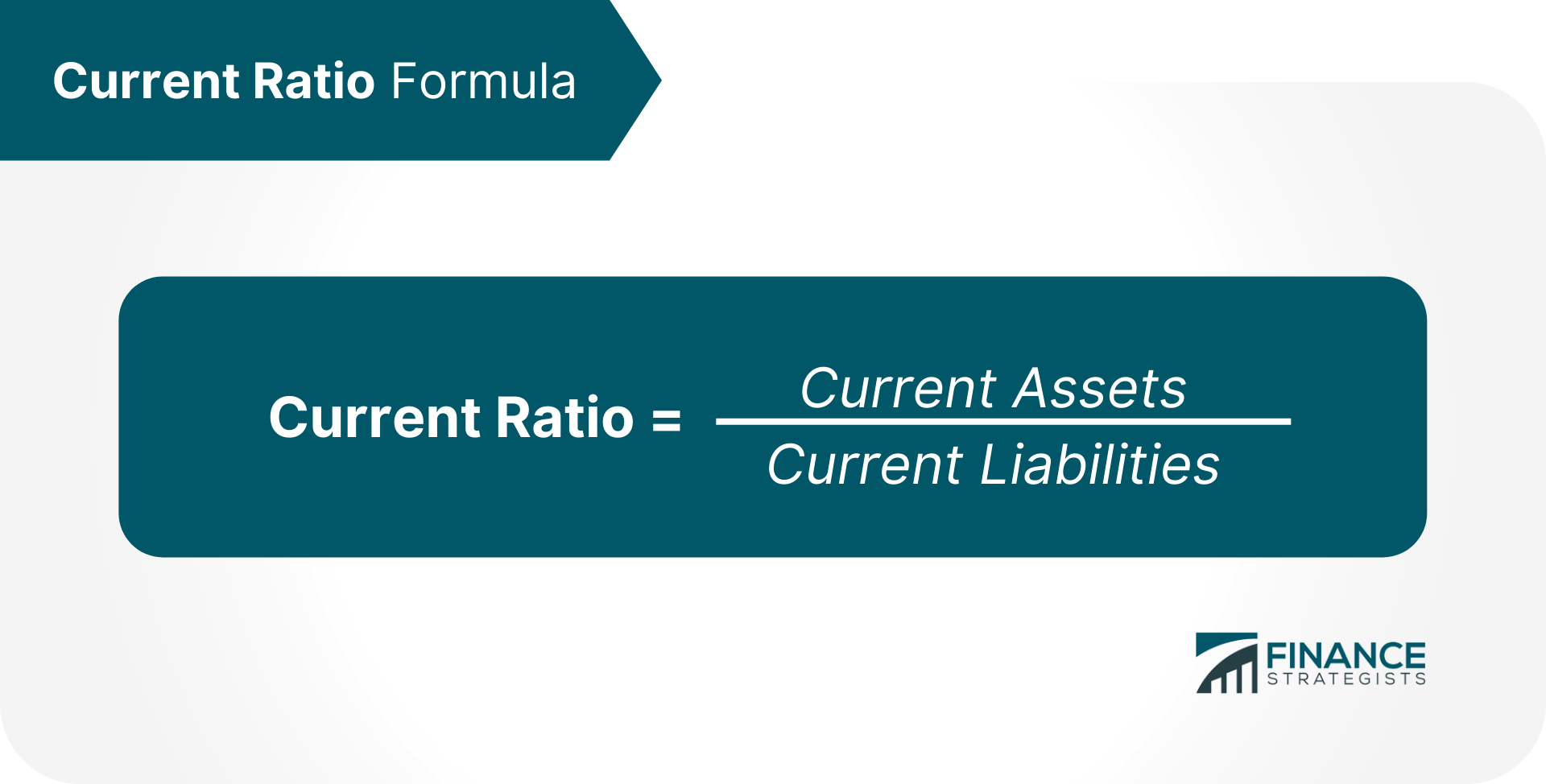# Current Ratio### Written byTrue Tamplin, BSc, CEPF® | Reviewed by Editorial Team

Updated on December 20, 2022

## Current Ratio Definition

The current ratio is a liquidity ratio that is used to calculate a company's ability to meet its short-term debt and obligations, or those due in a single year, using assets available on its balance sheet.

It is also known as working capital ratio. A current ratio of one or more is preferred by investors.
A current ratio less than one is an indicator that the company may not be able to service its short-term debt.

On the other hand, a current ratio greater than one can also be a sign that the company has too much unsold inventory or cash on hand.

## Current Ratio Formula## Current Ratio Calculation

Current assets refers to the sum of all assets that will be used or turned to cash in the next year.

This list includes cash, inventory, and accounts receivables. Current liabilities refers to the sum of all liabilities that are due in the next year.
This list includes wages, accounts payable and mortgage payments and loans.

For example, if a company has \$100,000 in current assets and \$150,000 in current liabilities, then its current ratio is 0.6.

## What Does the Current Ratio Measure?

Current ratios can vary depending on industry, size of company, and economic conditions.

Industries with predictable, recurring revenue, such as consumer goods, often have lower current ratios while cyclical industries, such as construction, have high current ratios. Even within an industry, current ratios can differ between companies.

For example, supplier agreements can make a difference to the number of liabilities and assets. A large retailer like Walmart may negotiate favorable terms with suppliers that allow it to keep inventory for longer periods and have generous payment terms or liabilities.

## What Is a Good Current Ratio?

During times of economic growth, investors prefer lean companies with low current ratios and ask for dividends from companies with high current ratios. But, during recessions, they flock to companies with high current ratios because they have current assets that can help weather downturns.

Current ratios are not always a good snapshot of company liquidity because they assume that all inventory and assets can be immediately converted to cash. This may not always be the case, especially during economic recessions.

In such cases, acid-test ratios are used because they subtract inventory from asset calculations to calculate immediate liquidity.

## Current Ratio FAQs

### What is the Current Ratio?

The current ratio is a liquidity ratio that is used to calculate a company’s ability to meet its short-term debt and obligations, or those due in a single year, using assets available on its balance sheet.

### What is the formula for the Current Ratio?

The formula for the current ratio is: Current Ratio = Current Assets / Current Liabilities

### What is a good current ratio?

A current ratio of one or more is preferred by investors.

### What's an example of current ratio?

For example, if a company has \$100,000 in current assets and \$150,000 in current liabilities, then its current ratio is 0.6.

### How reliable is the current ratio?

Current ratios are not always a good snapshot of company liquidity because they assume that all inventory and assets can be immediately converted to cash. This may not always be the case, especially during economic recessions. In such cases, acid-test ratios are used because they subtract inventory from asset calculations to calculate immediate liquidity.